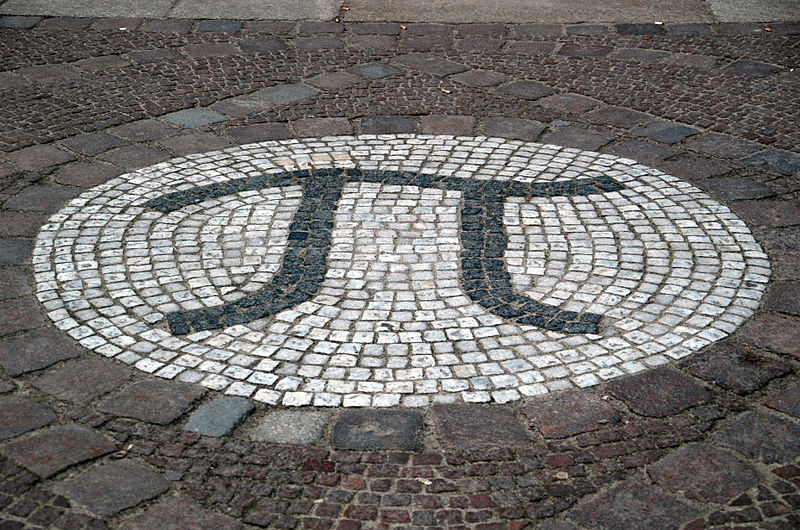National Pi Day 2018

March 14 may be just an ordinary day for you, but it is celebrated as Pi Day across the world to recognize the mathematical constant (3.14159 . . .) represented by π.

Pi is the ratio of a circle's circumference  (the length of the curved line that forms the circle) to its diameter (any straight line from one point on the circumference of the circle to another that passes through its center). The formula that connects them is C = 2πR, where C is the circumference and R is the radius (the straight line between the center of a circle to its circumference and exactly half of the diameter).

### Who started Pi Day?The Pi symbolWikimedia Commons

Larry Shaw organized the first Pi Day in 1988 at the Exploratorium Museum in San Francisco. Shaw worked as a physicist there. The staff celebrated the first happy Pi day eating fruit pies and maths lovers started following the trend. March 14 is also the birthday of Albert Einstein.

Another reason for selecting March 14 as Pi Day is that the numerical date (3.14) represents the first three digits of pi, reports the history.com.

In 2009, US House of Representatives passed legislation on March 12 and recognised March 14 as National Pi Day.

Now, Pi Day is celebrated by eating and throwing pies and discussing the significances of the recurring number.

### Why Pi Day?

But what is so special about Pi? Ancient Greek mathematician Archimedes is believed to be the first person to accurately calculate the estimated value of Pi. Though it is used in many mathematical formulae, we have not been able to calculate its exact value.

However, this never-ending number has a great significance in mathematics because of what it stands for in relation to a circle — it's, as mentioned, the constant ratio of a circle's circumference to its diameter. Engineers are also being able to make modern construction because of the pi.

Mathematicians, scientists and teachers are hoping the special day will increase people's interest in math and science, according to the website.